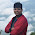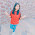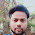» » Program Check even or odd without using modulus and division operators

# Program Check even or odd without using modulus and division operators

• Checking the number even or odd program is very easy. Anybody can solve this but there is a condition we need to see.
• When we get this question in interview "write a program to check given number is even or odd" always continues with "without using modulus and division operators".
• Before going to actual program lets see how to check a number is even or odd by using modulus and division operators.

#### Program to check number is even or odd by using modulus "%" operator

1. package instanceofjava;
2. import java.util.Scanner;
3.
4. public class EvenorOdd {
5.
6. public static void main(String []args )    {
7.
8.     int number;
9.     Scanner in= new Scanner(System.in);
10.
11.     System.out.println("Enter a number to check even or odd");
12.     number=in.nextInt();
13.
14.     if((number % 2)==0){
15.         System.out.println(+number+" is Even number");
16.     }else{
17.         System.out.println(+number+" is Odd Number");
18.     }
19.
20. }
21. }

#### Output:

1. Enter a number to check even or odd
2. 37
3. 37 is Odd Number

#### Program to check number is even or odd by using division "/" operator

1. package instanceofjava;
2. import java.util.Scanner;
3.
4. public class EvenorOdd {
5.
6. public static void main(String []args )    {
7.
8.     int number;
9.     Scanner in= new Scanner(System.in);
10.
11.     System.out.println("Enter a number to check even or odd");
12.     number=in.nextInt();
13.
14.     if((number / 2)*2==number){
15.         System.out.println(+number+" is Even number");
16.     }else{
17.         System.out.println(+number+" is Odd Number");
18.     }
19.
20. }
21. }

#### Output:

1. Enter a number to check even or odd
2. 46
3. 46 is Even Number

### Without using modulus and division operators:

• The above two programs will check number is even or odd and displays result.
• Now we need to write a program to check even or odd without using modulus and division operators.
• It is very simple if you know about operators including "BIT WISE".
• Yes using Bit Wise AND "&" operator we can check a number is even or odd.
• Before starting our program lets see how this bit wise AND "&" operator will work.

### Bitwise Operators :

• Bit wise operators will work on bits at a time.
• AND : 1 & 1=1
• OR :     0 | 1= 1 , 1 | 0=1 , 1| 1= 1
• XOR:   0 ^ 1= 1 , 1^ 0=1
• NOT : !0=1
• Take two number 2 and 3
• 010 : 2
011 : 3
------
010 : 2
• ------
• Take two numbers 2 and 1
• 010  :2
001  :1
-----
000  :0
-----
• From above example we can say that on every even number & 1 gives 0.
• So this is our logic to be implemented in our program  if "Number & 1==0" then its even number.

#### Program to check number is even or odd by using  "&" operator

1. package instanceofjava;
2. import java.util.Scanner;
3.
4. public class EvenorOdd {
5.
6. public static void main(String []args )    {
7.
8.     int number;
9.     Scanner in= new Scanner(System.in);
10.
11.     System.out.println("Enter a number to check even or odd");
12.     number=in.nextInt();
13.
14.     if((number & 1)==0){
15.         System.out.println(+number+" is Even number");
16.     }else{
17.         System.out.println(+number+" is Odd Number");
18.     }
19.
20. }
21. }

#### Output:

1. Enter a number to check even or odd
2. 9
3. 9 is Odd Number

### Using Shift Operators:

• We can check even or odd by using shift operators also may be it is not a better solution but  trying to cover alternative.
• Lets see how shift operators will work
• Lets do this 2 >> 1
• 2 means 010 so now we need to shift right side by 1 place
• 010
-----
001
• Here we added one "0" to the left side to shift right
• So the value became 1
• Now will try left shift  001 >> 1
001
----
010
• 010<<1=001(1) and 001 >> 001=010(2)
• By this we can say if a (number >>1)<<1 gives same then that is even number. result=input
• Lets check for odd number 3
• 011>>1=001 which is 1 and 001<<1=010 which is 2 so (3>>1)>>1=2 so result!=input

#### Program to check number is even or odd by using Shift operator

1. package instanceofjava;
2. import java.util.Scanner;
3.
4. public class EvenorOdd {
5.
6. public static void main(String []args )    {
7.
8.     int number;
9.     Scanner in= new Scanner(System.in);
10.
11.     System.out.println("Enter a number to check even or odd");
12.     number=in.nextInt();
13.
14.     if( ( number >> 1) <<1==number){
15.         System.out.println(+number+" is Even number");
16.     }else{
17.         System.out.println(+number+" is Odd Number");
18.     }
19.
20. }
21. }

#### Output:

1. Enter a number to check even or odd
2. 64
3. 64 is Even Number
Here is the another solution for this.
1. package instanceofjava;
2. import java.util.Scanner;
3.
4. class EvenOrOddDemo
5. {
6.
7. public static void main(String[] args)
8. {
9.
10. Scanner sc=new Scanner(System.in);
11.
12. System.out.println("Enter a number to check whether it is even or odd without using Modulus
13. and Division: ");
14.
15. int n=Integer.parseInt(sc.next());
16. float f=(float)n/(float)2;
17. int d=(int)f*2;
18.
19. if(d==n)
20. System.out.println(n+" is a even number");
21. else
22. System.out.println(n+" is a odd number");
23. }

24. }

#### Output:

1. Enter a number to check whether it is even or odd without using Modulus and Division
2. 4
3. 4 is Even Number

You might like:

#### Instance Of Java

We will help you in learning.Please leave your comments and suggestions in comment section. if you any doubts please use search box provided right side. Search there for answers thank you.

#### 9 comments for Program Check even or odd without using modulus and division operators

1.good explanation

2.Very good with various method and explanations

3.Oh my god I have to review those operations very quickly :D

4.import java.util.Scanner;
class OddEven
{
public static void main(String args[])
{
int no;
Scanner s=new Scanner(System.in);
System.out.println("Enter any number : ");
no=s.nextInt();
if(no>0)
while(no!=0)
{
if(no==1)
{
System.out.println("Number is odd.");
return;
}
no-=2;
}
else
while(no!=0)
{
if(no==1)
{
System.out.println("Number is odd.");
return;
}
no+=2;
}
System.out.println("Number is even.");

// or

/*while(no!=0)
{
if(no==1)
{
System.out.println("Number is odd.");
return;
}
no+=2;
}
System.out.println("Number is even.");*/
}
}

5.Awsssmmmmmm......!!

6.I have one more solution.

import java.util.Scanner;
class OddEven
{
public static void main(String args[])
{
int no;
Scanner s=new Scanner(System.in);
System.out.println("Enter any number : ");
no=s.nextInt();
if(no>0)
while(no!=0)
{
if(no==1)
{
System.out.println("Number is odd.");
return;
}
no-=2;
}
else
while(no!=0)
{
if(no==1)
{
System.out.println("Number is odd.");
return;
}
no+=2;
}
System.out.println("Number is even.");

// or

/*while(no!=0)
{
if(no==1)
{
System.out.println("Number is odd.");
return;
}
no+=2;
}
System.out.println("Number is even.");*/
}
}

7.this article very helpfull

8.XOR is ^ in JAVA

9.good experiences